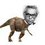# An algebra question from pre RMO 2015

Let $a,b,c$ be reals such that $a-7b+8c=4$ and $8a+4b-c=7$

Then find the value of $a^2-b^2+c^2$Note by Nihar Mahajan
5 years, 8 months ago

This discussion board is a place to discuss our Daily Challenges and the math and science related to those challenges. Explanations are more than just a solution — they should explain the steps and thinking strategies that you used to obtain the solution. Comments should further the discussion of math and science.

When posting on Brilliant:

• Use the emojis to react to an explanation, whether you're congratulating a job well done , or just really confused .
• Ask specific questions about the challenge or the steps in somebody's explanation. Well-posed questions can add a lot to the discussion, but posting "I don't understand!" doesn't help anyone.
• Try to contribute something new to the discussion, whether it is an extension, generalization or other idea related to the challenge.

MarkdownAppears as
*italics* or _italics_ italics
**bold** or __bold__ bold
- bulleted- list
• bulleted
• list
1. numbered2. list
1. numbered
2. list
Note: you must add a full line of space before and after lists for them to show up correctly
paragraph 1paragraph 2

paragraph 1

paragraph 2

[example link](https://brilliant.org)example link
> This is a quote
This is a quote
    # I indented these lines
# 4 spaces, and now they show
# up as a code block.

print "hello world"
# I indented these lines
# 4 spaces, and now they show
# up as a code block.

print "hello world"
MathAppears as
Remember to wrap math in $$ ... $$ or $ ... $ to ensure proper formatting.
2 \times 3 $2 \times 3$
2^{34} $2^{34}$
a_{i-1} $a_{i-1}$
\frac{2}{3} $\frac{2}{3}$
\sqrt{2} $\sqrt{2}$
\sum_{i=1}^3 $\sum_{i=1}^3$
\sin \theta $\sin \theta$
\boxed{123} $\boxed{123}$

Sort by:

Denote the two equations $(1)$ and $(2)$ respectively:

$(1)-2(2)$ gives us $a+b=\frac {2}{3}(1+c)$

$2(1)+(2)$ gives us $a-b=\frac {3}{2}(1-c)$

So $a^2-b^2+c^2=(a+b)(a-b)+c^2=1-c^2+c^2=1$.

- 5 years, 8 months ago

Nice question

Cryptic Hint: $65 = 5 \times 13$. These are both primes of the form $1 \pmod{4}$.

Staff - 5 years, 8 months ago

Don't you mean "of the form $1 \pmod{4}$"?

- 5 years, 8 months ago

Oooops, yes I do. Edited. Thanks!

Staff - 5 years, 8 months ago

Sir, can you explain how you got this? I'm sorry I do not know this method. :)

- 5 years, 8 months ago

Direct Hint: $65 = 1^2 + 8^2 = 7^2 + 4^2$

Explanation of cryptic hint: By Fermat's Two square theorem, since $65 = 5 \times 13 = ( 2^2 + 1^2 ) ( 2^2 + 3^2 )$, we can write 65 as the sum of two (positive) squares in two distinct ways.

Staff - 5 years, 8 months ago

Wow! Nice way!

- 5 years, 8 months ago

We can use matrices to find the values of a,b,c

- 5 years, 8 months ago

You have 3 variables and 2 equations, so you do not have a completely determined system.

Though, the claim is that $a^2 - b^2 + c^2$ is independent of the actual values. The point of the question is to prove that the expression is always equal to 1.

Staff - 5 years, 8 months ago

I don't know whether this method is valid , but we learn from mistakes and hence, I try:

Computing for $b$ from both the equations,

$b=\dfrac{a+8c-4}{7}=\dfrac{7+c-8a}{4}$

Now simplifying the two equations at the right , we get $12a+5c=13\Rightarrow 12(13a)+5(13c)=13^2$ which imitates the Pythagorean triplet $12^2+5^2=13^2$ and then comparing them we have $13a=12 \ , \ 13c=5$ which when substituted in the either of the first equations , we have $b=0$. Now substituting the acquired values in required expression we have $a^2-b^2+c^2=1$.

This solution is incomplete since it assumes that $13a,13c$ are integers.

- 5 years, 8 months ago

This method doesn't constitute a proof, since you're essentially substituting $b =0 , a = \frac{12}{13}, c = \frac{5}{13}$.

If you want a proof by substitution, you could
1. Determine $a$ and $b$ in terms of $c$ only.
2. Substitute these values into $a^2 - b^2 + c^ 2$.
3. Hope that everything cancels out (which it should, if your arithmetic is correct).

Staff - 5 years, 8 months ago

Slightly easier approach that follows from my hint. Consider:

$(a-7b+8c)^2 + (8a+4b-c)^2$

Notice that the cross terms cancel out.

This exploits the symmetry of the values, and the observation that $1^2 + 8^2 = 4^2 + 7^2$.

Staff - 5 years, 8 months ago

2^{0}

- 3 years ago

0

- 2 years, 12 months ago

Eliminating $a,b,c$ one by one from both equations we get 3 equations:

$13a+5b=12$ ...1

$13c-12b=5$ ...2

$12a-5c=13$ ...3

Again by elimination of each of $a,b$ and $c$,we get $a=\frac{13}{12},b=-\frac{5}{12},c=0$

Putting them in $a^{2}-b^{2}+c^{2}$ we get $\boxed{1}$.

- 5 years, 8 months ago

It is wrong to simply assume that $c=0$. We must show that the answer is independent of $c$.

- 5 years, 8 months ago